# MSBSHSE Solutions For Class 8 Maths Part 1 Chapter 5- Expansion Formulae

MSBSHSE Solutions For Class 8 Maths Part 1 Chapter 5 – Expansion Formulae are readily available here. This Chapter mainly deals with expansion of different forms of expressions. Mathematics is one of the scoring subjects where students secure maximum marks in the exam. When it comes to preparing for the exam, it is the toughest time where most students struggle to solve problems. So, here at BYJU’S, our expert faculty team have developed Maharashtra State Board Class 8 Textbooks Part 1 solutions, which help students effortlessly prepare for their exams. All the solutions are well designed, keeping in mind the latest Maharashtra Board patterns and guidelines. Students can also learn easy tricks and short cut techniques when practised on a regular basis. The PDF of Maharashtra Board Solutions for Class 8 Maths Chapter 5 Expansion Formulae is available here, which students can download for free, from the links mentioned.

## Download the PDF of Maharashtra Board Solutions For Class 8 Maths Part 1 Chapter 5- Expansion Formulae.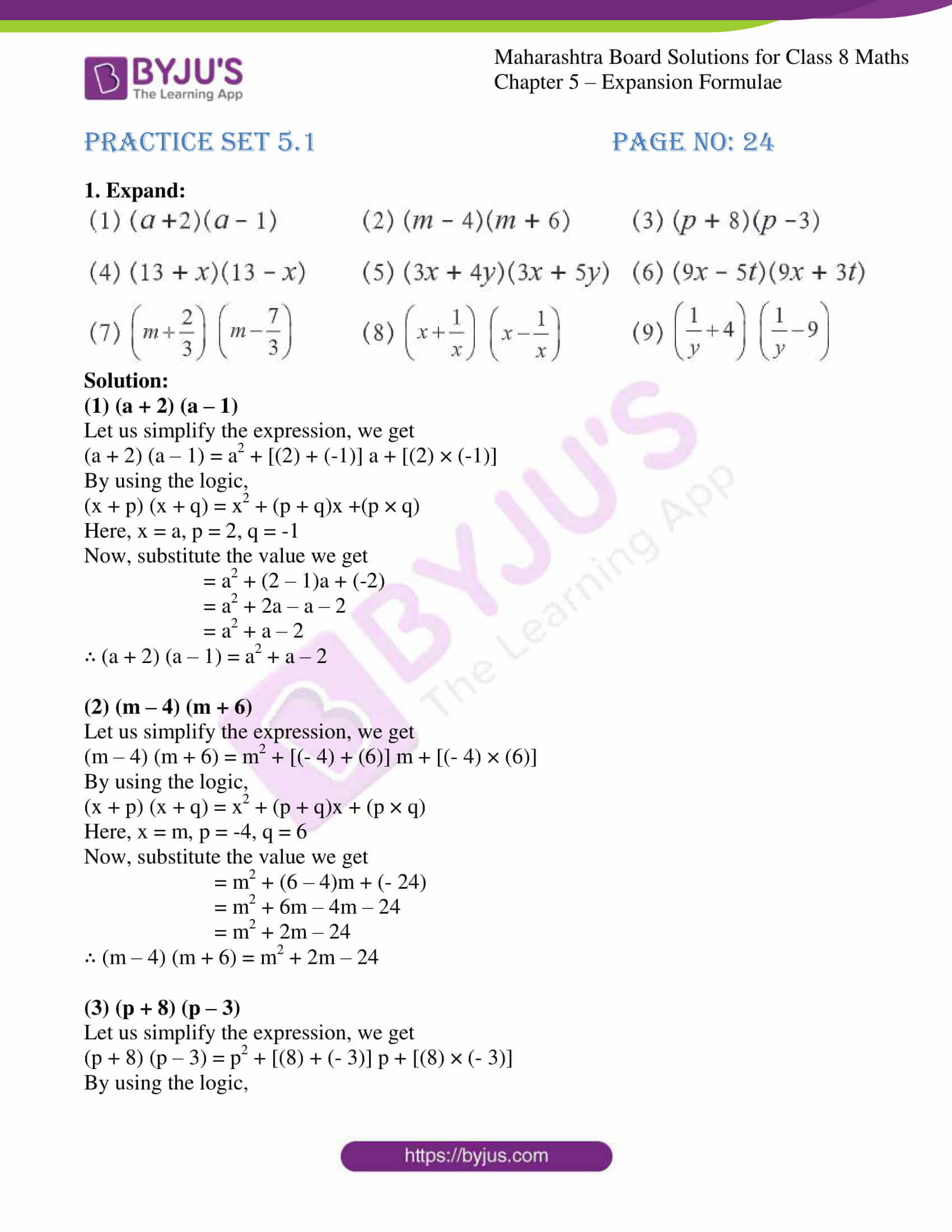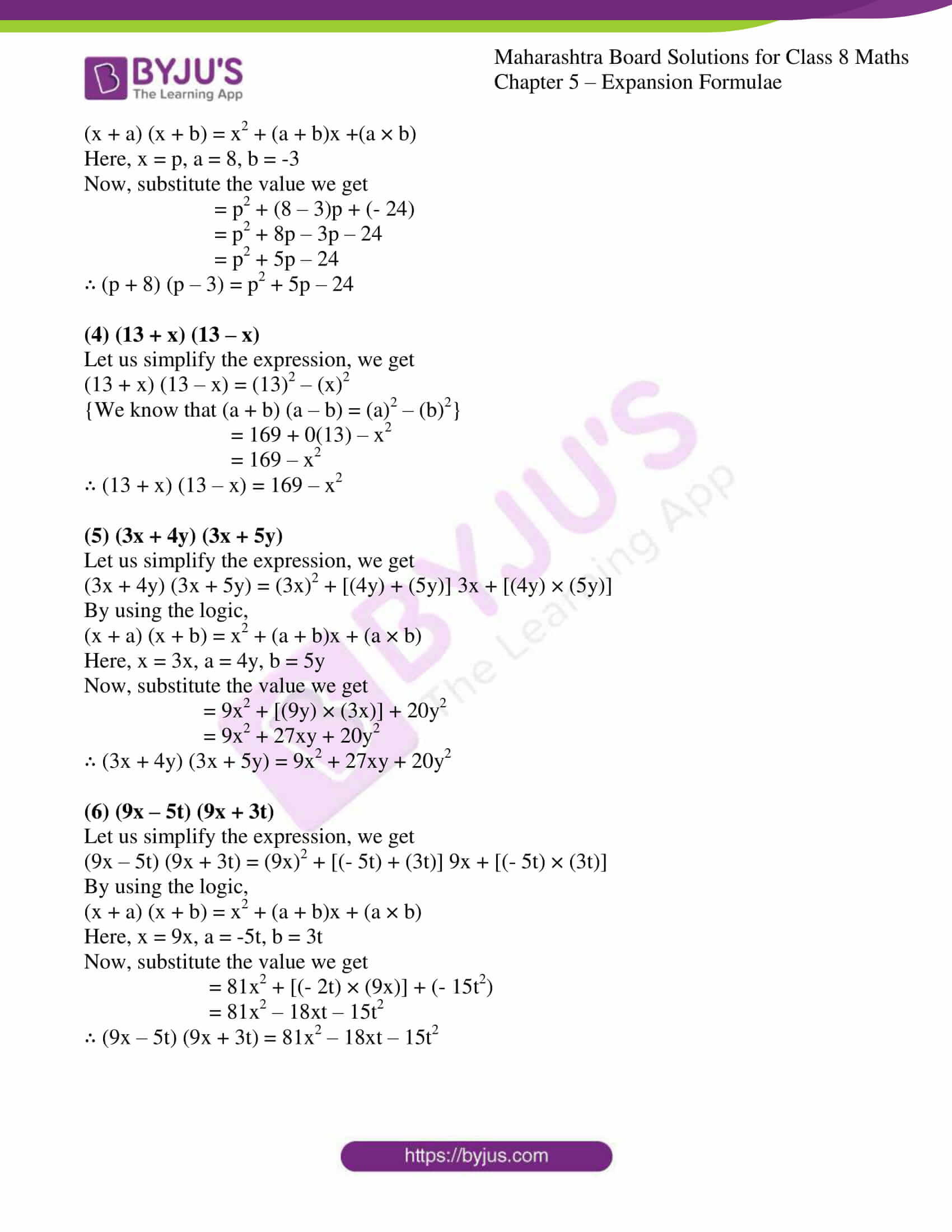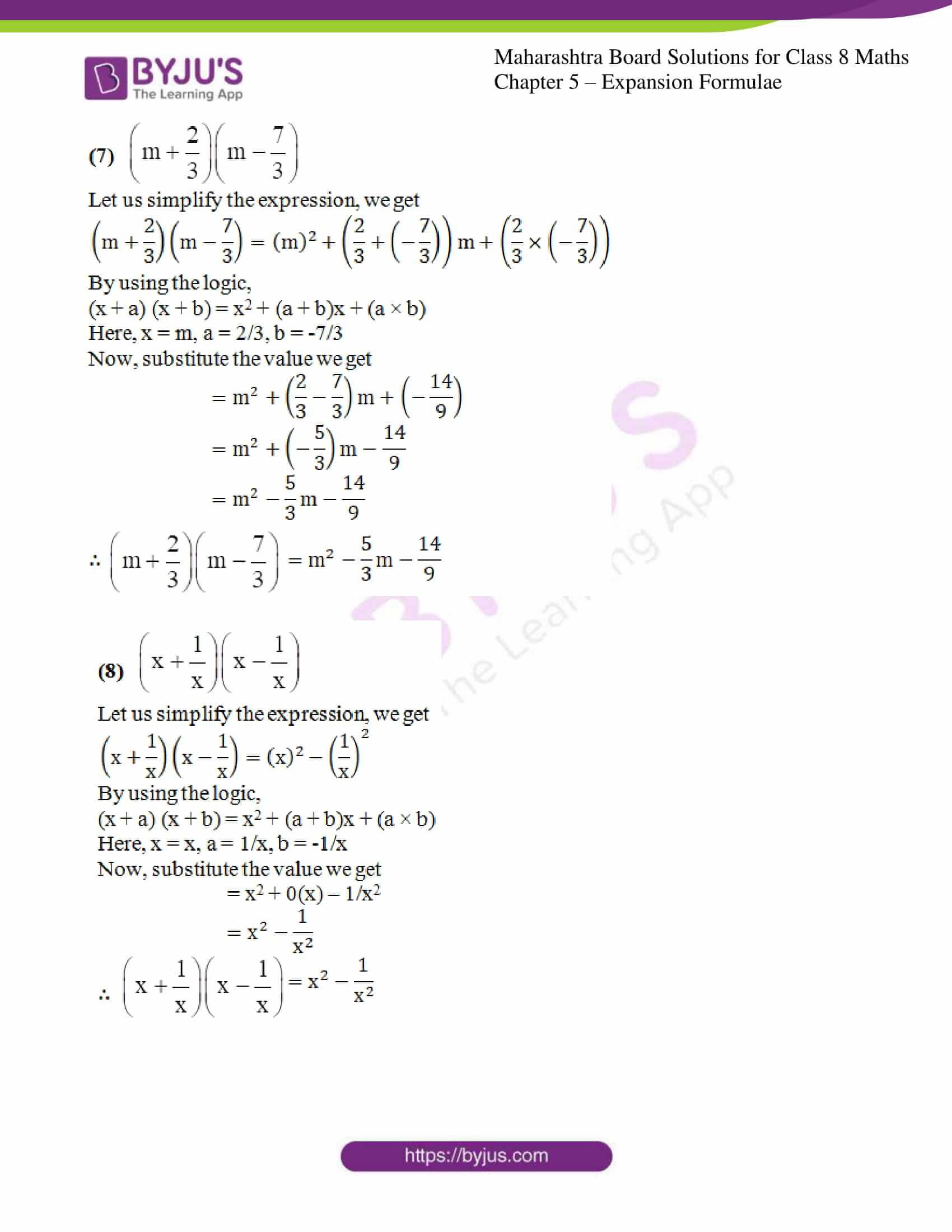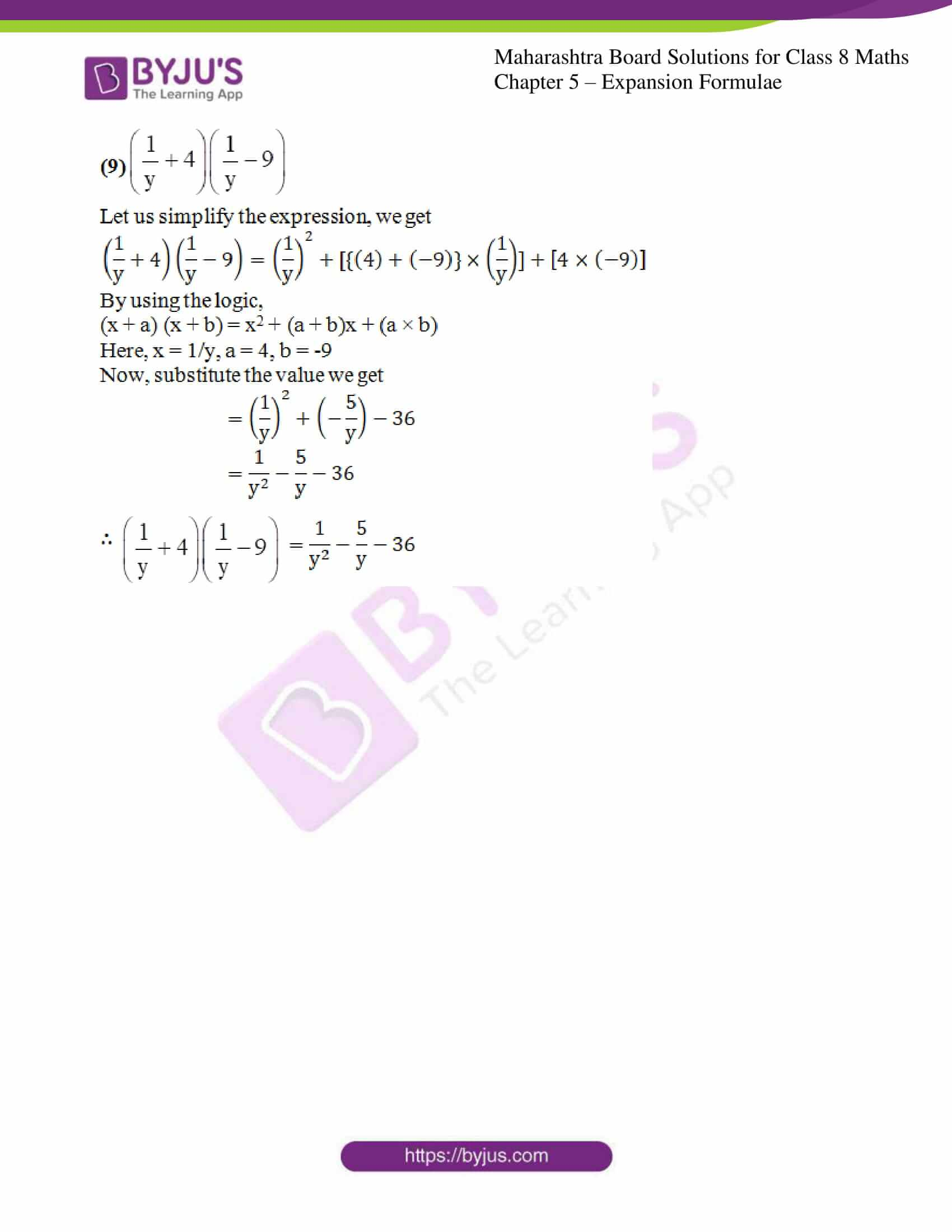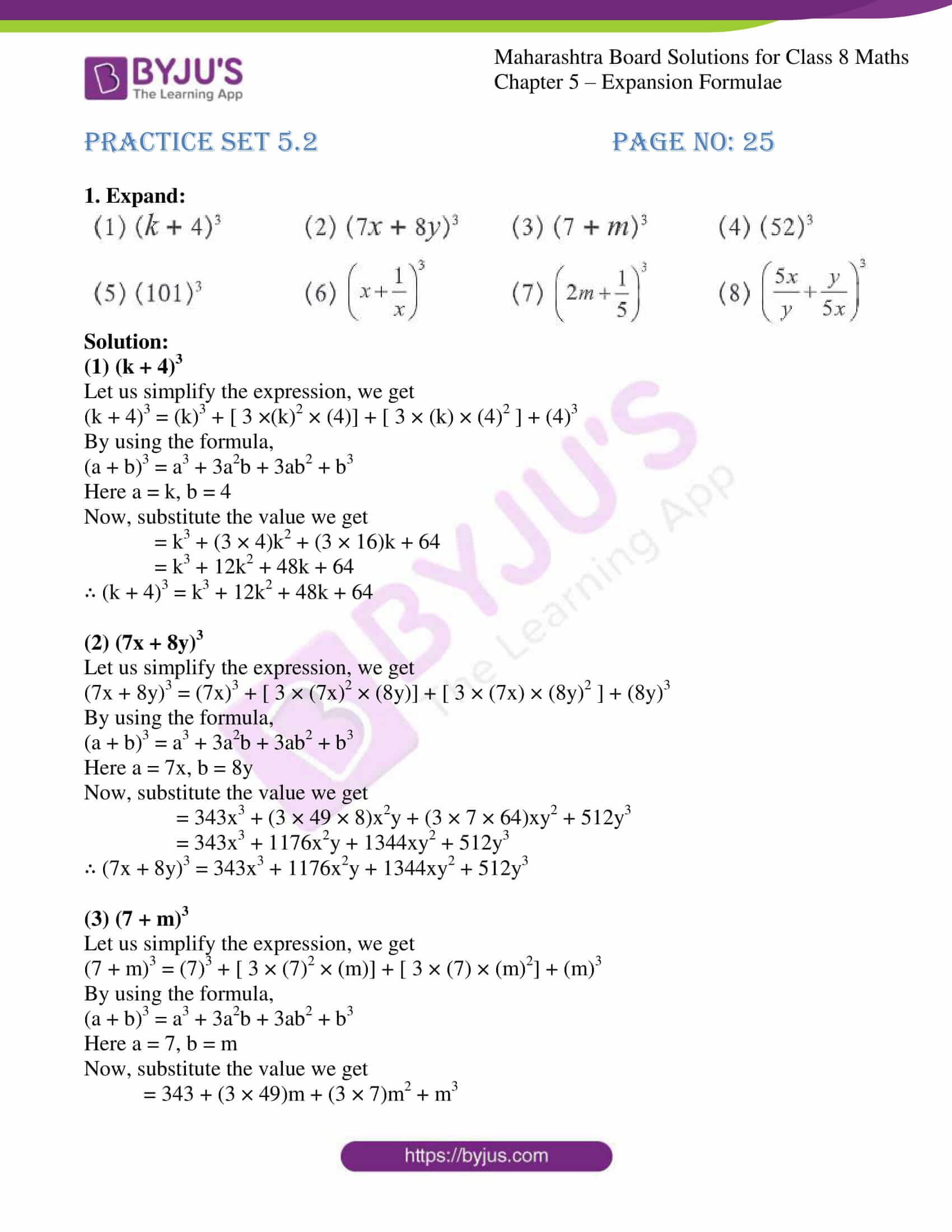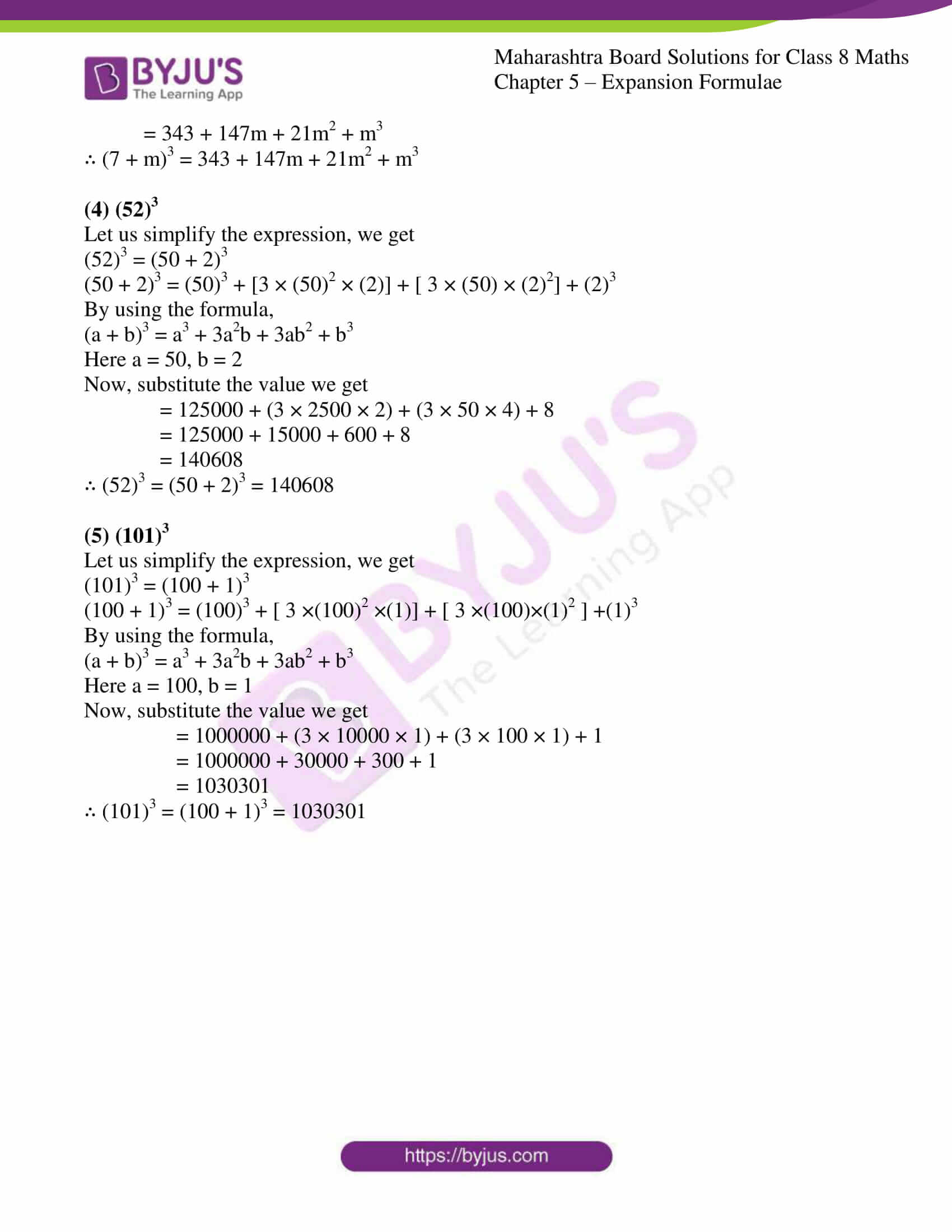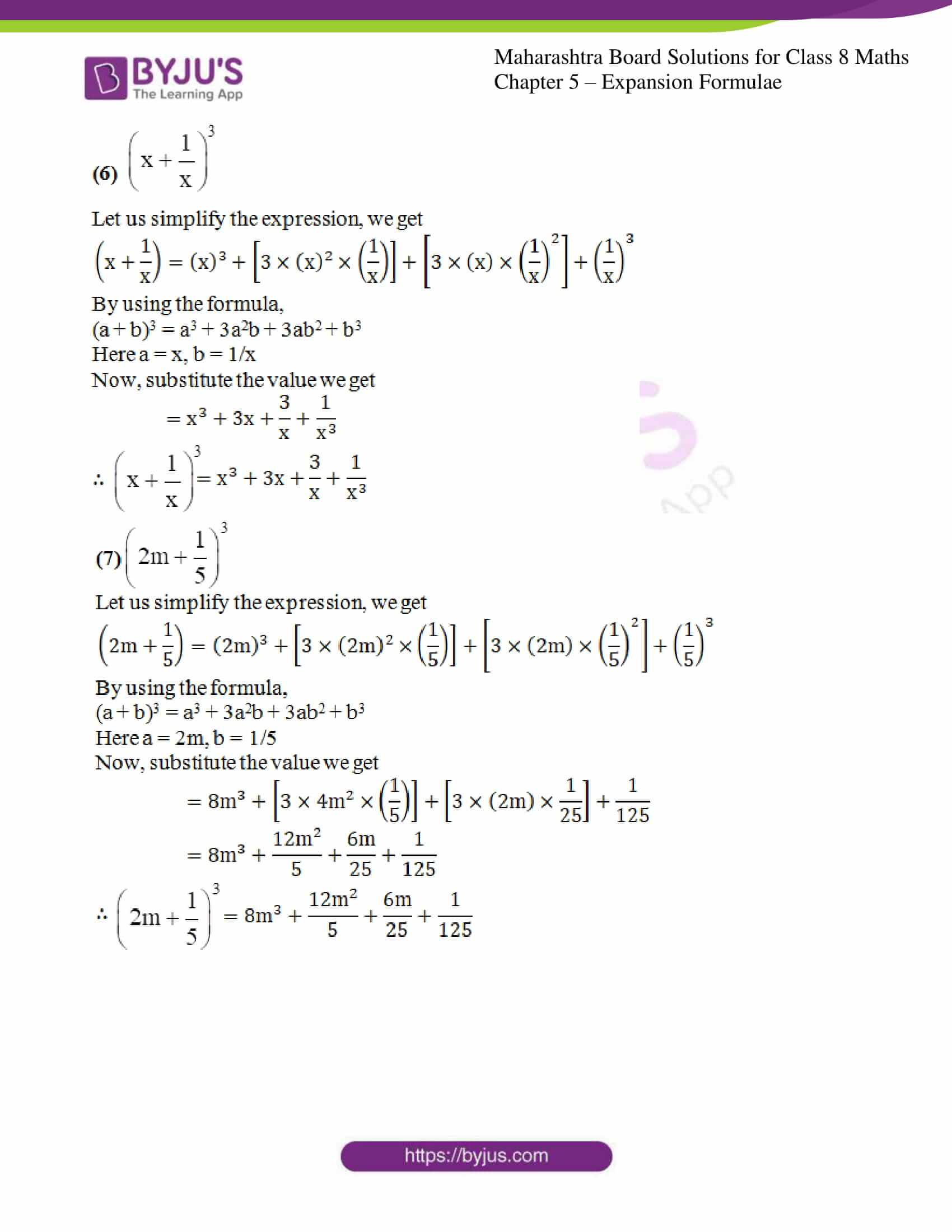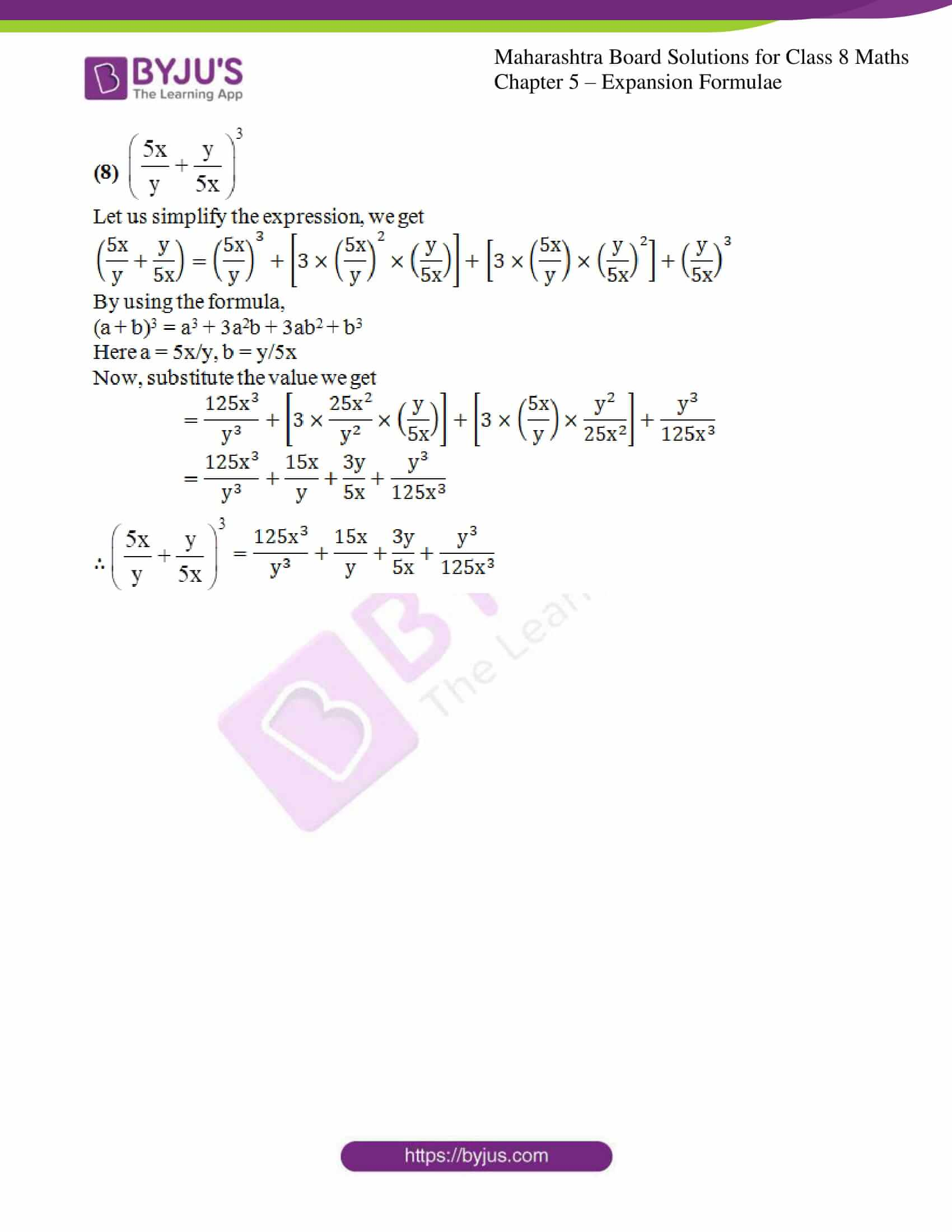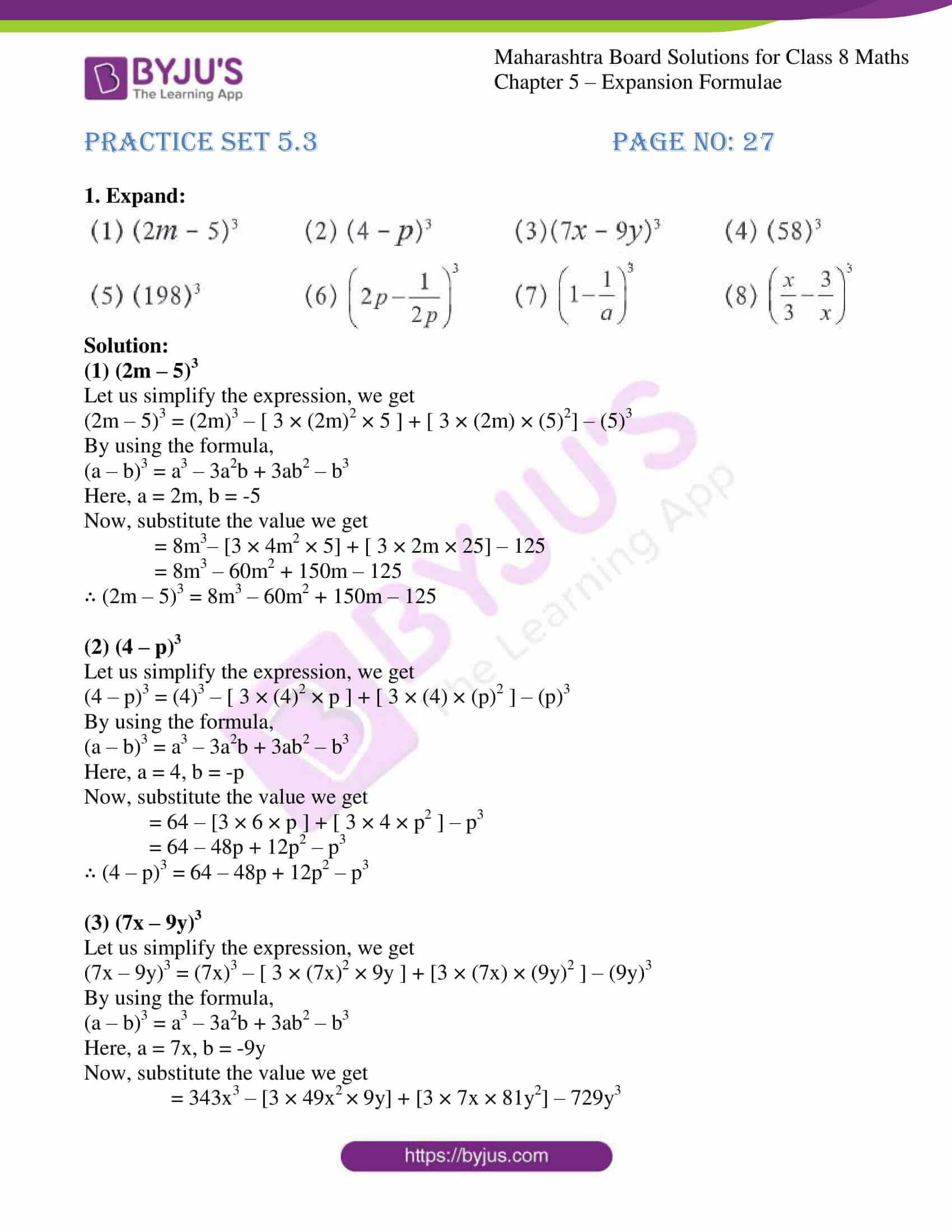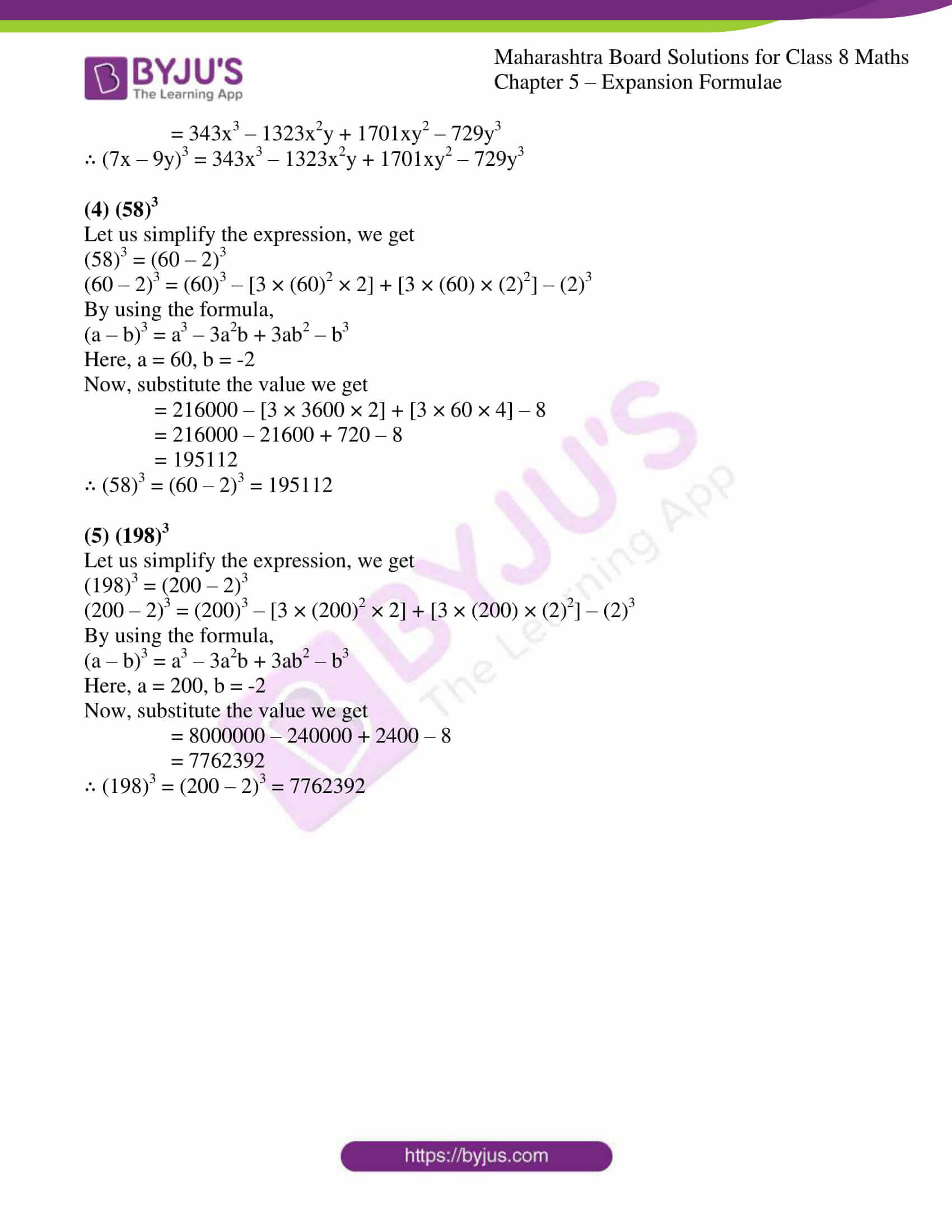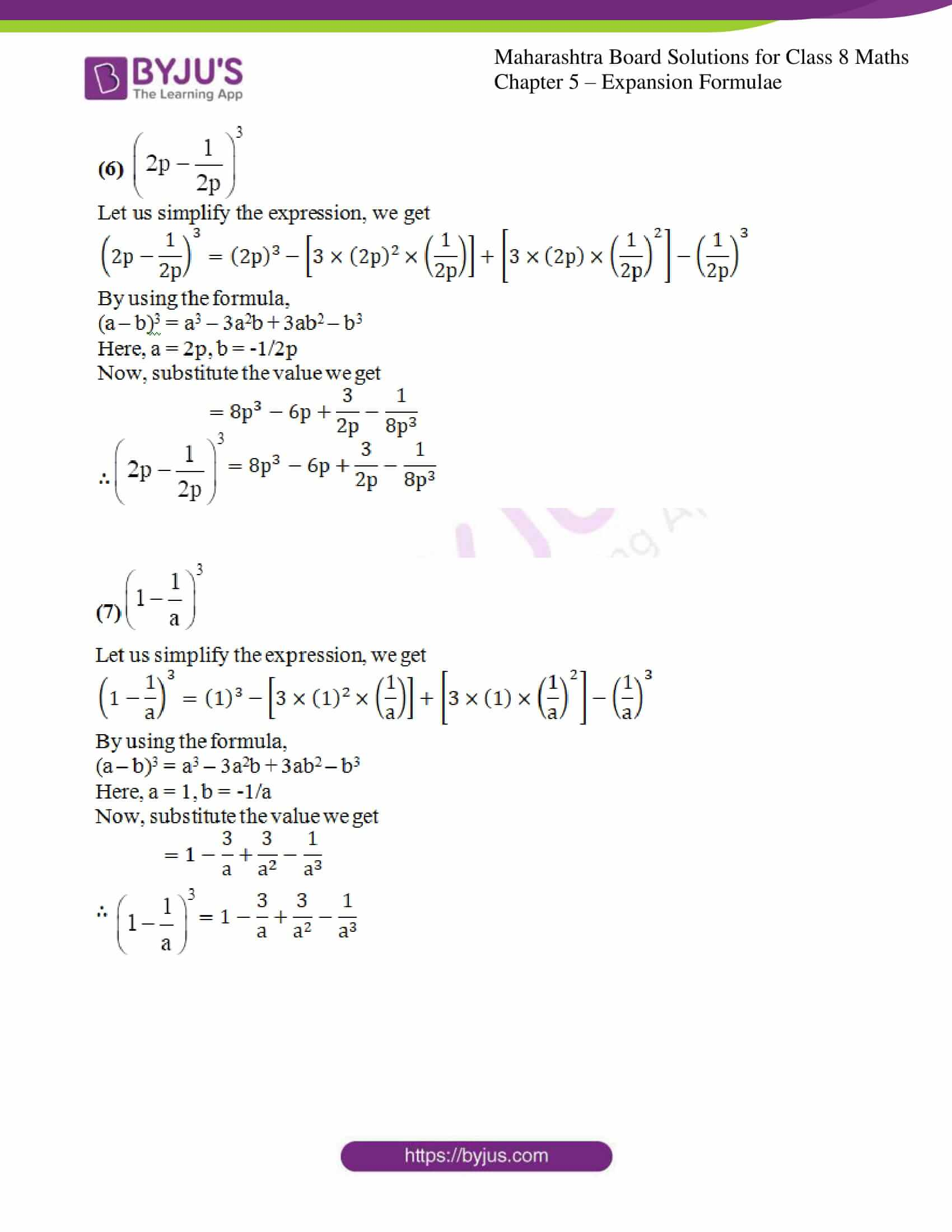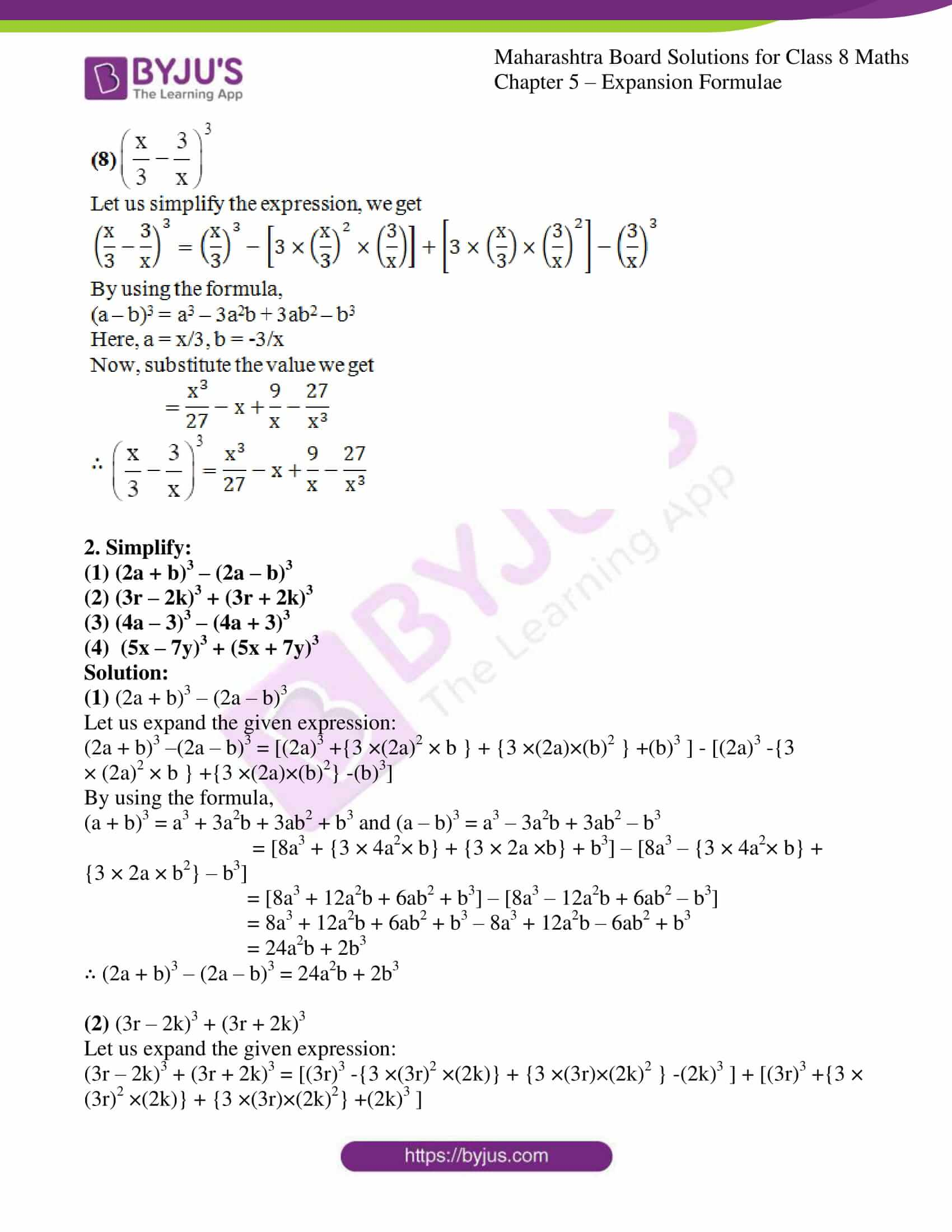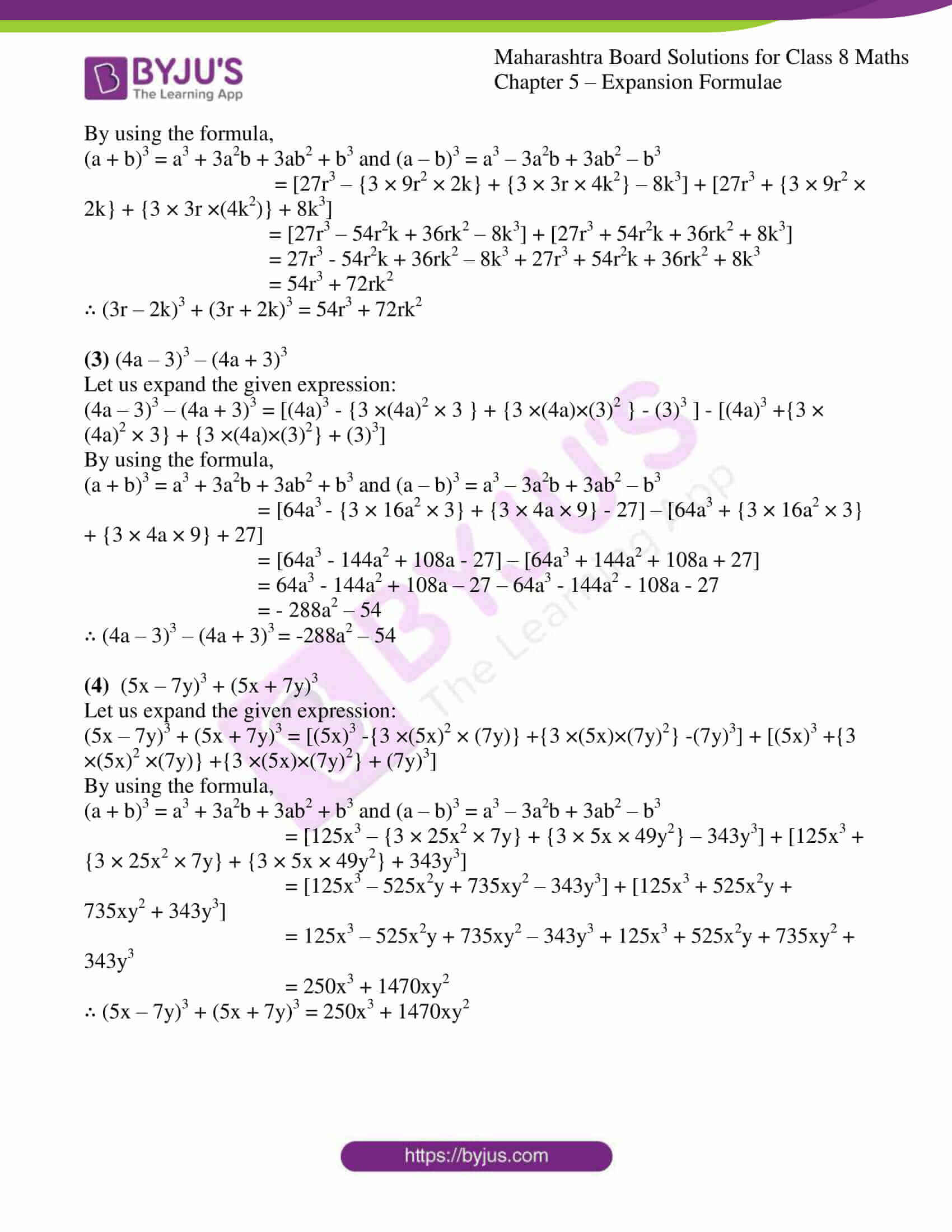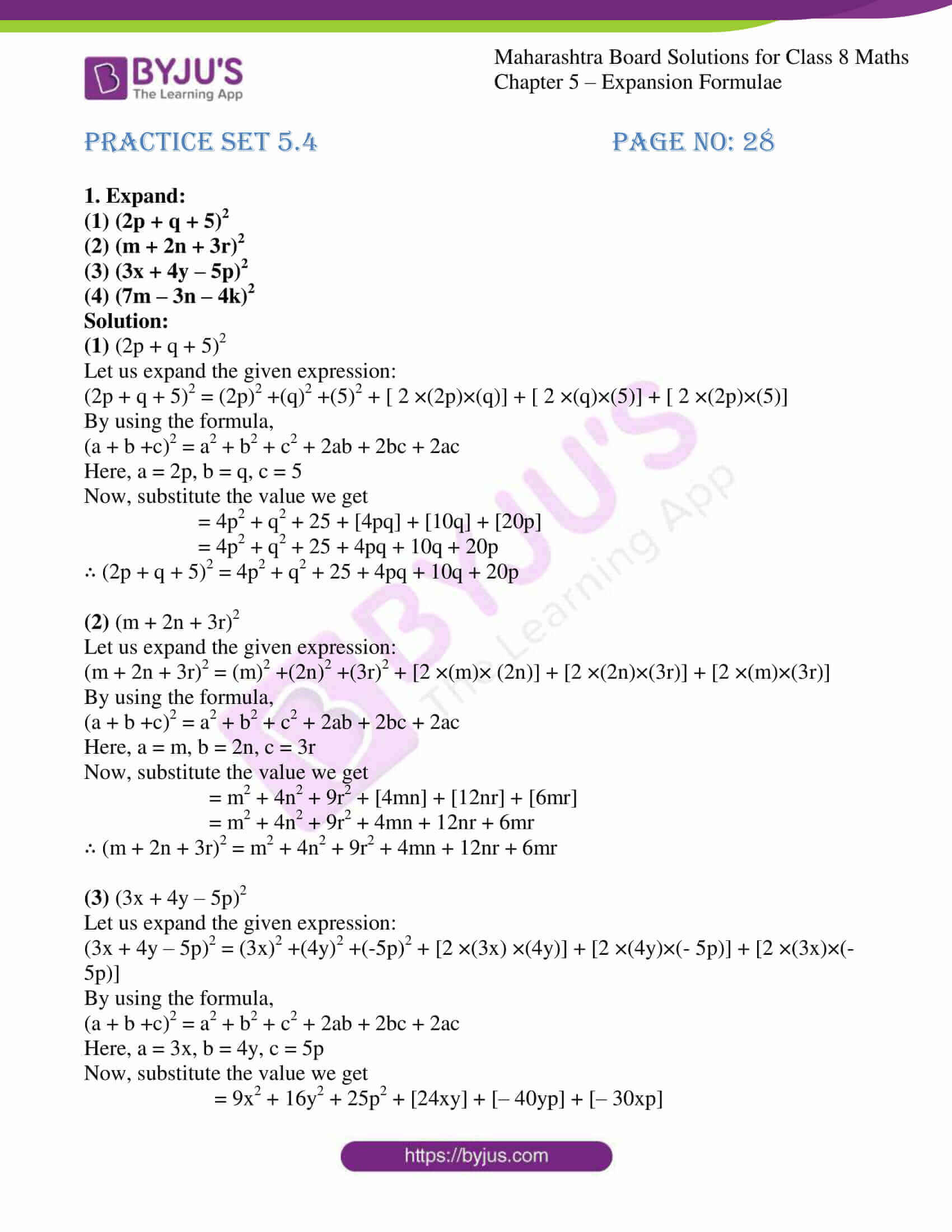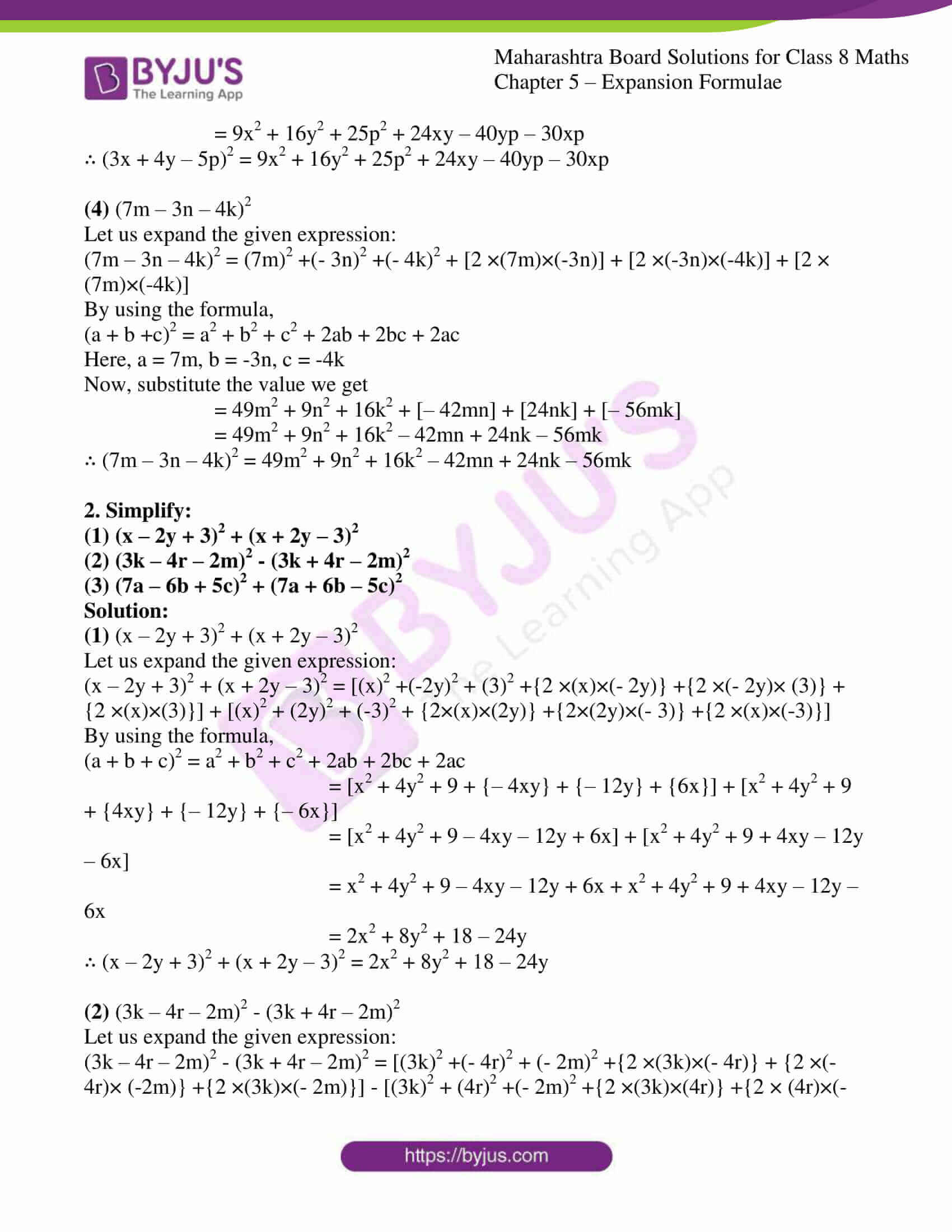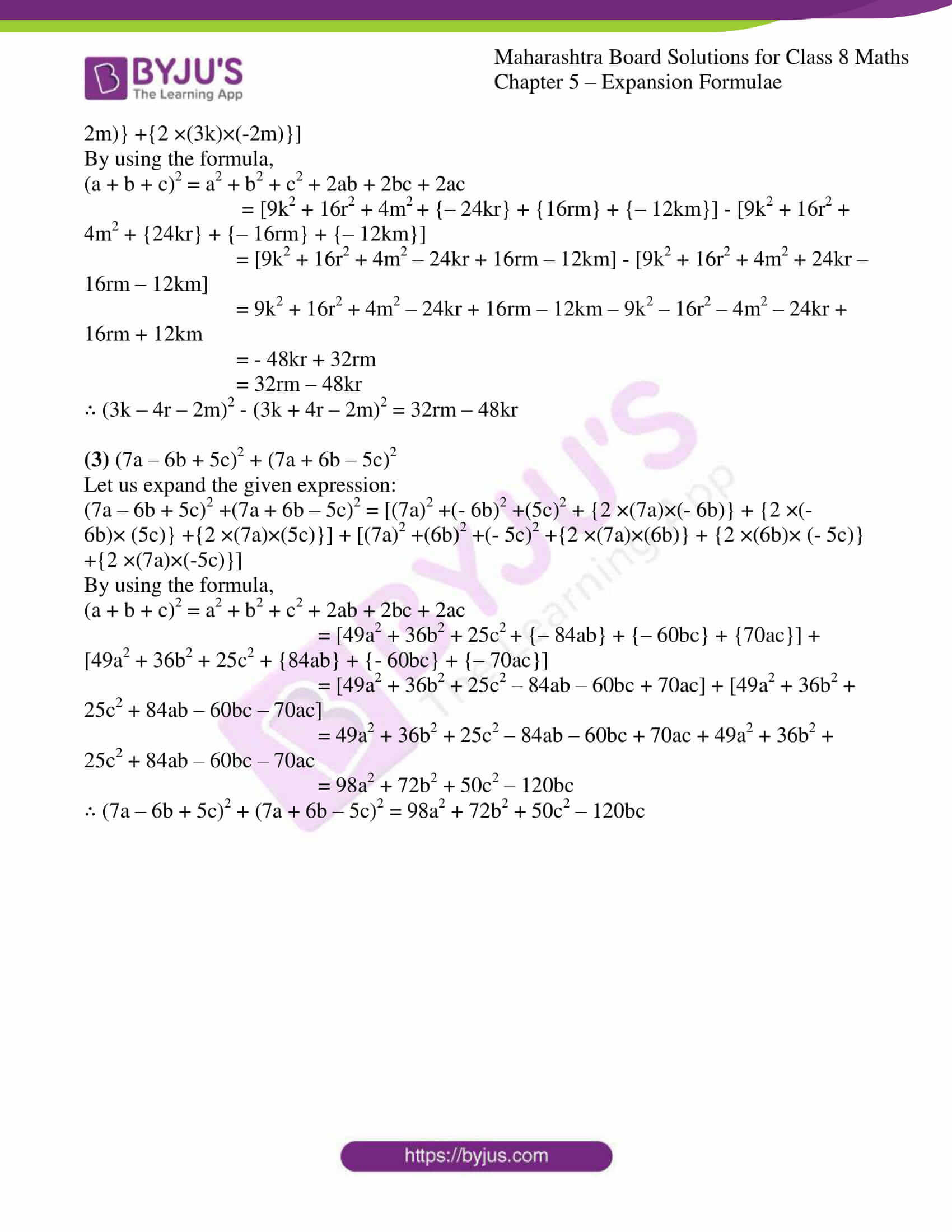### Access answers to Maharashtra Board Solutions For Class 8 Maths Part 1 Chapter 5- Expansion Formulae.

Practice set 5.1 PAGE NO: 24

1. Expand: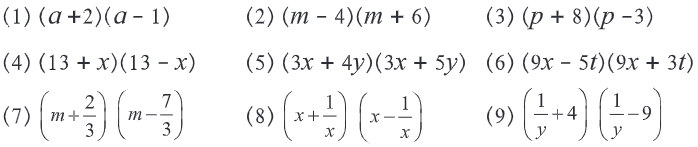Solution:

(1) (a + 2) (a – 1)

Let us simplify the expression, we get

(a + 2) (a – 1) = a2 + [(2) + (-1)] a + [(2) × (-1)]

By using the logic,

(x + p) (x + q) = x2 + (p + q)x +(p × q)

Here, x = a, p = 2, q = -1

Now, substitute the value we get

= a2 + (2 – 1)a + (-2)

= a2 + 2a – a – 2

= a2 + a – 2

∴ (a + 2) (a – 1) = a2 + a – 2

(2) (m – 4) (m + 6)

Let us simplify the expression, we get

(m – 4) (m + 6) = m2 + [(- 4) + (6)] m + [(- 4) × (6)]

By using the logic,

(x + p) (x + q) = x2 + (p + q)x + (p × q)

Here, x = m, p = -4, q = 6

Now, substitute the value we get

= m2 + (6 – 4)m + (- 24)

= m2 + 6m – 4m – 24

= m2 + 2m – 24

∴ (m – 4) (m + 6) = m2 + 2m – 24

(3) (p + 8) (p – 3)

Let us simplify the expression, we get

(p + 8) (p – 3) = p2 + [(8) + (- 3)] p + [(8) × (- 3)]

By using the logic,

(x + a) (x + b) = x2 + (a + b)x +(a × b)

Here, x = p, a = 8, b = -3

Now, substitute the value we get

= p2 + (8 – 3)p + (- 24)

= p2 + 8p – 3p – 24

= p2 + 5p – 24

∴ (p + 8) (p – 3) = p2 + 5p – 24

(4) (13 + x) (13 – x)

Let us simplify the expression, we get

(13 + x) (13 – x) = (13)2 – (x)2

{We know that (a + b) (a – b) = (a)2 – (b)2}

= 169 + 0(13) – x2

= 169 – x2

∴ (13 + x) (13 – x) = 169 – x2

(5) (3x + 4y) (3x + 5y)

Let us simplify the expression, we get

(3x + 4y) (3x + 5y) = (3x)2 + [(4y) + (5y)] 3x + [(4y) × (5y)]

By using the logic,

(x + a) (x + b) = x2 + (a + b)x + (a × b)

Here, x = 3x, a = 4y, b = 5y

Now, substitute the value we get

= 9x2 + [(9y) × (3x)] + 20y2

= 9x2 + 27xy + 20y2

∴ (3x + 4y) (3x + 5y) = 9x2 + 27xy + 20y2

(6) (9x – 5t) (9x + 3t)

Let us simplify the expression, we get

(9x – 5t) (9x + 3t) = (9x)2 + [(- 5t) + (3t)] 9x + [(- 5t) × (3t)]

By using the logic,

(x + a) (x + b) = x2 + (a + b)x + (a × b)

Here, x = 9x, a = -5t, b = 3t

Now, substitute the value we get

= 81x2 + [(- 2t) × (9x)] + (- 15t2)

= 81x2 – 18xt – 15t2

∴ (9x – 5t) (9x + 3t) = 81x2 – 18xt – 15t2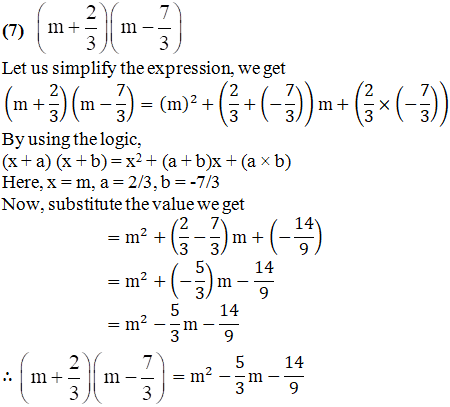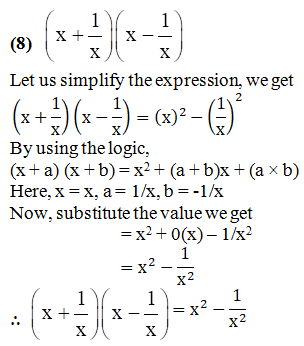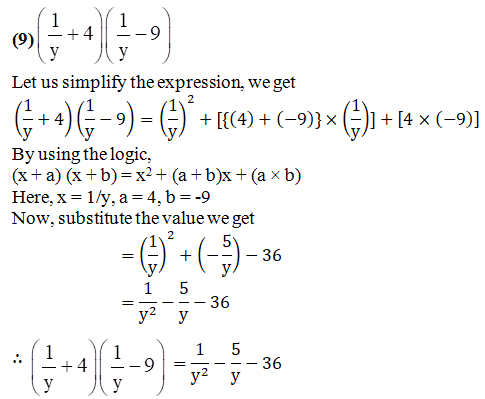Practice set 5.2 PAGE NO: 25

1. Expand: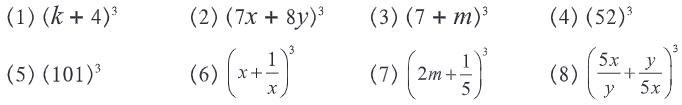Solution:

(1) (k + 4)3

Let us simplify the expression, we get

(k + 4)3 = (k)3 + [ 3 ×(k)2 × (4)] + [ 3 × (k) × (4)2 ] + (4)3

By using the formula,

(a + b)3 = a3 + 3a2b + 3ab2 + b3

Here a = k, b = 4

Now, substitute the value we get

= k3 + (3 × 4)k2 + (3 × 16)k + 64

= k3 + 12k2 + 48k + 64

∴ (k + 4)3 = k3 + 12k2 + 48k + 64

(2) (7x + 8y)3

Let us simplify the expression, we get

(7x + 8y)3 = (7x)3 + [ 3 × (7x)2 × (8y)] + [ 3 × (7x) × (8y)2 ] + (8y)3

By using the formula,

(a + b)3 = a3 + 3a2b + 3ab2 + b3

Here a = 7x, b = 8y

Now, substitute the value we get

= 343x3 + (3 × 49 × 8)x2y + (3 × 7 × 64)xy2 + 512y3

= 343x3 + 1176x2y + 1344xy2 + 512y3

∴ (7x + 8y)3 = 343x3 + 1176x2y + 1344xy2 + 512y3

(3) (7 + m)3

Let us simplify the expression, we get

(7 + m)3 = (7)3 + [ 3 × (7)2 × (m)] + [ 3 × (7) × (m)2] + (m)3

By using the formula,

(a + b)3 = a3 + 3a2b + 3ab2 + b3

Here a = 7, b = m

Now, substitute the value we get

= 343 + (3 × 49)m + (3 × 7)m2 + m3

= 343 + 147m + 21m2 + m3

∴ (7 + m)3 = 343 + 147m + 21m2 + m3

(4) (52)3

Let us simplify the expression, we get

(52)3 = (50 + 2)3

(50 + 2)3 = (50)3 + [3 × (50)2 × (2)] + [ 3 × (50) × (2)2] + (2)3

By using the formula,

(a + b)3 = a3 + 3a2b + 3ab2 + b3

Here a = 50, b = 2

Now, substitute the value we get

= 125000 + (3 × 2500 × 2) + (3 × 50 × 4) + 8

= 125000 + 15000 + 600 + 8

= 140608

∴ (52)3 = (50 + 2)3 = 140608

(5) (101)3

Let us simplify the expression, we get

(101)3 = (100 + 1)3

(100 + 1)3 = (100)3 + [ 3 ×(100)2 ×(1)] + [ 3 ×(100)×(1)2 ] +(1)3

By using the formula,

(a + b)3 = a3 + 3a2b + 3ab2 + b3

Here a = 100, b = 1

Now, substitute the value we get

= 1000000 + (3 × 10000 × 1) + (3 × 100 × 1) + 1

= 1000000 + 30000 + 300 + 1

= 1030301

∴ (101)3 = (100 + 1)3 = 1030301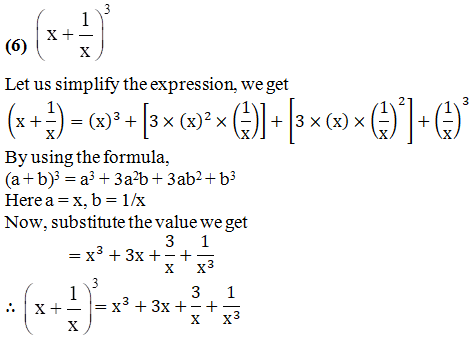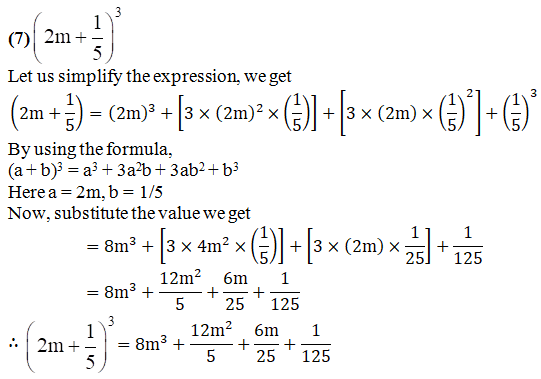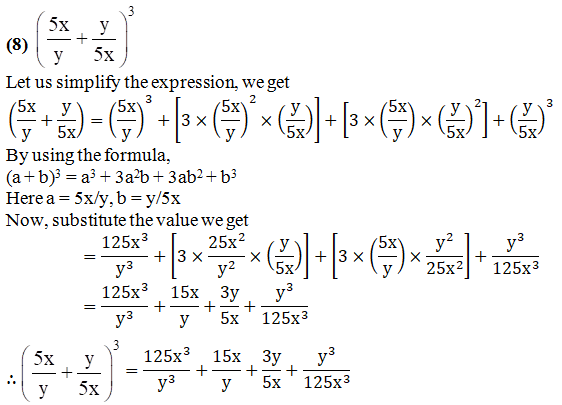Practice set 5.3 PAGE NO: 27

1. Expand: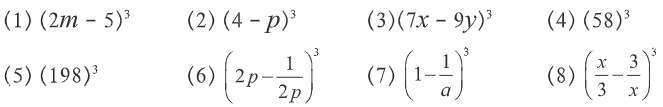Solution:

(1) (2m – 5)3

Let us simplify the expression, we get

(2m – 5)3 = (2m)3 – [ 3 × (2m)2 × 5 ] + [ 3 × (2m) × (5)2] – (5)3

By using the formula,

(a – b)3 = a3 – 3a2b + 3ab2 – b3

Here, a = 2m, b = -5

Now, substitute the value we get

= 8m3– [3 × 4m2 × 5] + [ 3 × 2m × 25] – 125

= 8m3 – 60m2 + 150m – 125

∴ (2m – 5)3 = 8m3 – 60m2 + 150m – 125

(2) (4 – p)3

Let us simplify the expression, we get

(4 – p)3 = (4)3 – [ 3 × (4)2 × p ] + [ 3 × (4) × (p)2 ] – (p)3

By using the formula,

(a – b)3 = a3 – 3a2b + 3ab2 – b3

Here, a = 4, b = -p

Now, substitute the value we get

= 64 – [3 × 6 × p ] + [ 3 × 4 × p2 ] – p3

= 64 – 48p + 12p2 – p3

∴ (4 – p)3 = 64 – 48p + 12p2 – p3

(3) (7x – 9y)3

Let us simplify the expression, we get

(7x – 9y)3 = (7x)3 – [ 3 × (7x)2 × 9y ] + [3 × (7x) × (9y)2 ] – (9y)3

By using the formula,

(a – b)3 = a3 – 3a2b + 3ab2 – b3

Here, a = 7x, b = -9y

Now, substitute the value we get

= 343x3 – [3 × 49x2 × 9y] + [3 × 7x × 81y2] – 729y3

= 343x3 – 1323x2y + 1701xy2 – 729y3

∴ (7x – 9y)3 = 343x3 – 1323x2y + 1701xy2 – 729y3

(4) (58)3

Let us simplify the expression, we get

(58)3 = (60 – 2)3

(60 – 2)3 = (60)3 – [3 × (60)2 × 2] + [3 × (60) × (2)2] – (2)3

By using the formula,

(a – b)3 = a3 – 3a2b + 3ab2 – b3

Here, a = 60, b = -2

Now, substitute the value we get

= 216000 – [3 × 3600 × 2] + [3 × 60 × 4] – 8

= 216000 – 21600 + 720 – 8

= 195112

∴ (58)3 = (60 – 2)3 = 195112

(5) (198)3

Let us simplify the expression, we get

(198)3 = (200 – 2)3

(200 – 2)3 = (200)3 – [3 × (200)2 × 2] + [3 × (200) × (2)2] – (2)3

By using the formula,

(a – b)3 = a3 – 3a2b + 3ab2 – b3

Here, a = 200, b = -2

Now, substitute the value we get

= 8000000 – 240000 + 2400 – 8

= 7762392

∴ (198)3 = (200 – 2)3 = 7762392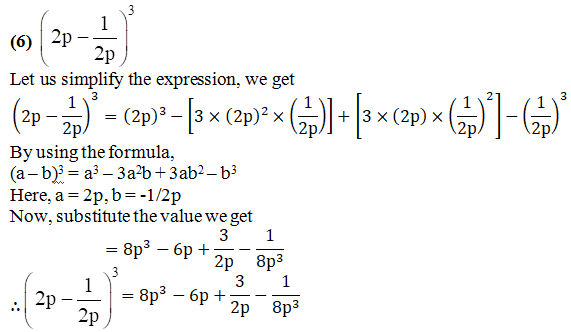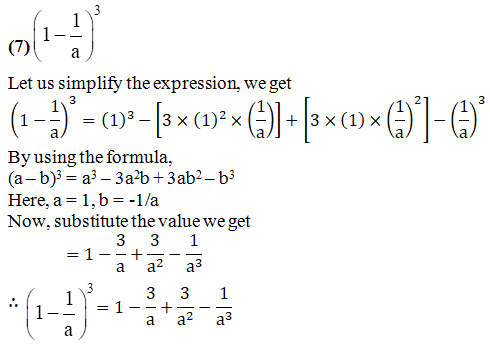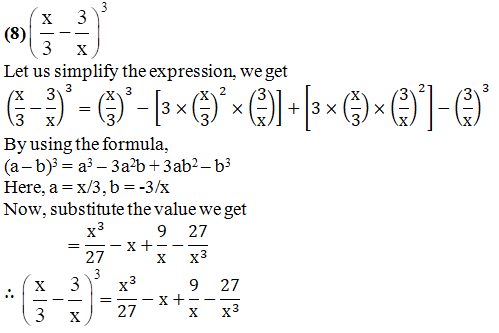2. Simplify:

(1) (2a + b)3 – (2a – b)3

(2) (3r – 2k)3 + (3r + 2k)3

(3) (4a – 3)3 – (4a + 3)3

(4) (5x – 7y)3 + (5x + 7y)3

Solution:

(1) (2a + b)3 – (2a – b)3

Let us expand the given expression:

(2a + b)3 –(2a – b)3 = [(2a)3 +{3 ×(2a)2 × b } + {3 ×(2a)×(b)2 } +(b)3 ] – [(2a)3 -{3 × (2a)2 × b } +{3 ×(2a)×(b)2} -(b)3]

By using the formula,

(a + b)3 = a3 + 3a2b + 3ab2 + b3 and (a – b)3 = a3 – 3a2b + 3ab2 – b3

= [8a3 + {3 × 4a2× b} + {3 × 2a ×b} + b3] – [8a3 – {3 × 4a2× b} + {3 × 2a × b2} – b3]

= [8a3 + 12a2b + 6ab2 + b3] – [8a3 – 12a2b + 6ab2 – b3]

= 8a3 + 12a2b + 6ab2 + b3 – 8a3 + 12a2b – 6ab2 + b3

= 24a2b + 2b3

∴ (2a + b)3 – (2a – b)3 = 24a2b + 2b3

(2) (3r – 2k)3 + (3r + 2k)3

Let us expand the given expression:

(3r – 2k)3 + (3r + 2k)3 = [(3r)3 -{3 ×(3r)2 ×(2k)} + {3 ×(3r)×(2k)2 } -(2k)3 ] + [(3r)3 +{3 × (3r)2 ×(2k)} + {3 ×(3r)×(2k)2} +(2k)3 ]

By using the formula,

(a + b)3 = a3 + 3a2b + 3ab2 + b3 and (a – b)3 = a3 – 3a2b + 3ab2 – b3

= [27r3 – {3 × 9r2 × 2k} + {3 × 3r × 4k2} – 8k3] + [27r3 + {3 × 9r2 × 2k} + {3 × 3r ×(4k2)} + 8k3]

= [27r3 – 54r2k + 36rk2 – 8k3] + [27r3 + 54r2k + 36rk2 + 8k3]

= 27r3 – 54r2k + 36rk2 – 8k3 + 27r3 + 54r2k + 36rk2 + 8k3

= 54r3 + 72rk2

∴ (3r – 2k)3 + (3r + 2k)3 = 54r3 + 72rk2

(3) (4a – 3)3 – (4a + 3)3

Let us expand the given expression:

(4a – 3)3 – (4a + 3)3 = [(4a)3 – {3 ×(4a)2 × 3 } + {3 ×(4a)×(3)2 } – (3)3 ] – [(4a)3 +{3 × (4a)2 × 3} + {3 ×(4a)×(3)2} + (3)3]

By using the formula,

(a + b)3 = a3 + 3a2b + 3ab2 + b3 and (a – b)3 = a3 – 3a2b + 3ab2 – b3

= [64a3 – {3 × 16a2 × 3} + {3 × 4a × 9} – 27] – [64a3 + {3 × 16a2 × 3} + {3 × 4a × 9} + 27]

= [64a3 – 144a2 + 108a – 27] – [64a3 + 144a2 + 108a + 27]

= 64a3 – 144a2 + 108a – 27 – 64a3 – 144a2 – 108a – 27

= – 288a2 – 54

∴ (4a – 3)3 – (4a + 3)3 = -288a2 – 54

(4) (5x – 7y)3 + (5x + 7y)3

Let us expand the given expression:

(5x – 7y)3 + (5x + 7y)3 = [(5x)3 -{3 ×(5x)2 × (7y)} +{3 ×(5x)×(7y)2} -(7y)3] + [(5x)3 +{3 ×(5x)2 ×(7y)} +{3 ×(5x)×(7y)2} + (7y)3]

By using the formula,

(a + b)3 = a3 + 3a2b + 3ab2 + b3 and (a – b)3 = a3 – 3a2b + 3ab2 – b3

= [125x3 – {3 × 25x2 × 7y} + {3 × 5x × 49y2} – 343y3] + [125x3 + {3 × 25x2 × 7y} + {3 × 5x × 49y2} + 343y3]

= [125x3 – 525x2y + 735xy2 – 343y3] + [125x3 + 525x2y + 735xy2 + 343y3]

= 125x3 – 525x2y + 735xy2 – 343y3 + 125x3 + 525x2y + 735xy2 + 343y3

= 250x3 + 1470xy2

∴ (5x – 7y)3 + (5x + 7y)3 = 250x3 + 1470xy2

Practice set 5.4 PAGE NO: 28

1. Expand:

(1) (2p + q + 5)2

(2) (m + 2n + 3r)2

(3) (3x + 4y – 5p)2

(4) (7m – 3n – 4k)2

Solution:

(1) (2p + q + 5)2

Let us expand the given expression:

(2p + q + 5)2 = (2p)2 +(q)2 +(5)2 + [ 2 ×(2p)×(q)] + [ 2 ×(q)×(5)] + [ 2 ×(2p)×(5)]

By using the formula,

(a + b +c)2 = a2 + b2 + c2 + 2ab + 2bc + 2ac

Here, a = 2p, b = q, c = 5

Now, substitute the value we get

= 4p2 + q2 + 25 + [4pq] + [10q] + [20p]

= 4p2 + q2 + 25 + 4pq + 10q + 20p

∴ (2p + q + 5)2 = 4p2 + q2 + 25 + 4pq + 10q + 20p

(2) (m + 2n + 3r)2

Let us expand the given expression:

(m + 2n + 3r)2 = (m)2 +(2n)2 +(3r)2 + [2 ×(m)× (2n)] + [2 ×(2n)×(3r)] + [2 ×(m)×(3r)]

By using the formula,

(a + b +c)2 = a2 + b2 + c2 + 2ab + 2bc + 2ac

Here, a = m, b = 2n, c = 3r

Now, substitute the value we get

= m2 + 4n2 + 9r2 + [4mn] + [12nr] + [6mr]

= m2 + 4n2 + 9r2 + 4mn + 12nr + 6mr

∴ (m + 2n + 3r)2 = m2 + 4n2 + 9r2 + 4mn + 12nr + 6mr

(3) (3x + 4y – 5p)2

Let us expand the given expression:

(3x + 4y – 5p)2 = (3x)2 +(4y)2 +(-5p)2 + [2 ×(3x) ×(4y)] + [2 ×(4y)×(- 5p)] + [2 ×(3x)×(-5p)]

By using the formula,

(a + b +c)2 = a2 + b2 + c2 + 2ab + 2bc + 2ac

Here, a = 3x, b = 4y, c = 5p

Now, substitute the value we get

= 9x2 + 16y2 + 25p2 + [24xy] + [– 40yp] + [– 30xp]

= 9x2 + 16y2 + 25p2 + 24xy – 40yp – 30xp

∴ (3x + 4y – 5p)2 = 9x2 + 16y2 + 25p2 + 24xy – 40yp – 30xp

(4) (7m – 3n – 4k)2

Let us expand the given expression:

(7m – 3n – 4k)2 = (7m)2 +(- 3n)2 +(- 4k)2 + [2 ×(7m)×(-3n)] + [2 ×(-3n)×(-4k)] + [2 × (7m)×(-4k)]

By using the formula,

(a + b +c)2 = a2 + b2 + c2 + 2ab + 2bc + 2ac

Here, a = 7m, b = -3n, c = -4k

Now, substitute the value we get

= 49m2 + 9n2 + 16k2 + [– 42mn] + [24nk] + [– 56mk]

= 49m2 + 9n2 + 16k2 – 42mn + 24nk – 56mk

∴ (7m – 3n – 4k)2 = 49m2 + 9n2 + 16k2 – 42mn + 24nk – 56mk

2. Simplify:

(1) (x – 2y + 3)2 + (x + 2y – 3)2

(2) (3k – 4r – 2m)2 – (3k + 4r – 2m)2

(3) (7a – 6b + 5c)2 + (7a + 6b – 5c)2

Solution:

(1) (x – 2y + 3)2 + (x + 2y – 3)2

Let us expand the given expression:

(x – 2y + 3)2 + (x + 2y – 3)2 = [(x)2 +(-2y)2 + (3)2 +{2 ×(x)×(- 2y)} +{2 ×(- 2y)× (3)} + {2 ×(x)×(3)}] + [(x)2 + (2y)2 + (-3)2 + {2×(x)×(2y)} +{2×(2y)×(- 3)} +{2 ×(x)×(-3)}]

By using the formula,

(a + b + c)2 = a2 + b2 + c2 + 2ab + 2bc + 2ac

= [x2 + 4y2 + 9 + {– 4xy} + {– 12y} + {6x}] + [x2 + 4y2 + 9 + {4xy} + {– 12y} + {– 6x}]

= [x2 + 4y2 + 9 – 4xy – 12y + 6x] + [x2 + 4y2 + 9 + 4xy – 12y – 6x]

= x2 + 4y2 + 9 – 4xy – 12y + 6x + x2 + 4y2 + 9 + 4xy – 12y – 6x

= 2x2 + 8y2 + 18 – 24y

∴ (x – 2y + 3)2 + (x + 2y – 3)2 = 2x2 + 8y2 + 18 – 24y

(2) (3k – 4r – 2m)2 – (3k + 4r – 2m)2

Let us expand the given expression:

(3k – 4r – 2m)2 – (3k + 4r – 2m)2 = [(3k)2 +(- 4r)2 + (- 2m)2 +{2 ×(3k)×(- 4r)} + {2 ×(- 4r)× (-2m)} +{2 ×(3k)×(- 2m)}] – [(3k)2 + (4r)2 +(- 2m)2 +{2 ×(3k)×(4r)} +{2 × (4r)×(- 2m)} +{2 ×(3k)×(-2m)}]

By using the formula,

(a + b + c)2 = a2 + b2 + c2 + 2ab + 2bc + 2ac

= [9k2 + 16r2 + 4m2 + {– 24kr} + {16rm} + {– 12km}] – [9k2 + 16r2 + 4m2 + {24kr} + {– 16rm} + {– 12km}]

= [9k2 + 16r2 + 4m2 – 24kr + 16rm – 12km] – [9k2 + 16r2 + 4m2 + 24kr – 16rm – 12km]

= 9k2 + 16r2 + 4m2 – 24kr + 16rm – 12km – 9k2 – 16r2 – 4m2 – 24kr + 16rm + 12km

= – 48kr + 32rm

= 32rm – 48kr

∴ (3k – 4r – 2m)2 – (3k + 4r – 2m)2 = 32rm – 48kr

(3) (7a – 6b + 5c)2 + (7a + 6b – 5c)2

Let us expand the given expression:

(7a – 6b + 5c)2 +(7a + 6b – 5c)2 = [(7a)2 +(- 6b)2 +(5c)2 + {2 ×(7a)×(- 6b)} + {2 ×(- 6b)× (5c)} +{2 ×(7a)×(5c)}] + [(7a)2 +(6b)2 +(- 5c)2 +{2 ×(7a)×(6b)} + {2 ×(6b)× (- 5c)} +{2 ×(7a)×(-5c)}]

By using the formula,

(a + b + c)2 = a2 + b2 + c2 + 2ab + 2bc + 2ac

= [49a2 + 36b2 + 25c2 + {– 84ab} + {– 60bc} + {70ac}] + [49a2 + 36b2 + 25c2 + {84ab} + {- 60bc} + {– 70ac}]

= [49a2 + 36b2 + 25c2 – 84ab – 60bc + 70ac] + [49a2 + 36b2 + 25c2 + 84ab – 60bc – 70ac]

= 49a2 + 36b2 + 25c2 – 84ab – 60bc + 70ac + 49a2 + 36b2 + 25c2 + 84ab – 60bc – 70ac

= 98a2 + 72b2 + 50c2 – 120bc

∴ (7a – 6b + 5c)2 + (7a + 6b – 5c)2 = 98a2 + 72b2 + 50c2 – 120bc

Our experts have simplified the difficult problems into simpler steps, which can be easily solved by students. These solutions will help you in obtaining knowledge and strong command over the subject. As the Chapter is about Expansion Formulae, regular revision of important concepts and formulas over time is the best way to strengthen your concepts.

Many such exercise problems are given in the MSBSHSE Solutions book, students can refer to them to yield good results in the examination.

## Frequently Asked Questions on Maharashtra State Board Solutions for Class 8 Maths Chapter 5 Expansion Formulae

### How are these Maharashtra State Board Class 8 Maths Chapter 5  Expansion Formulae Solutions helpful?

Students are encouraged to practise the questions and then to refer back to the solutions as it could help them analyse their performance. This will also help them to rectify the mistakes early on and avoid making any during the board exams.